Runners circle

Pepa circles the track in 36 seconds. Kamil in 42 seconds. They started together. How many seconds will meet again at the start?

Result

x =  252 s

Solution:Leave us a comment of this math problem and its solution (i.e. if it is still somewhat unclear...):Be the first to comment!To solve this verbal math problem are needed these knowledge from mathematics:

Do you want to calculate least common multiple two or more numbers?

Next similar math problems:

1. Bus linesBuses connections are started from bus stop on its regular circuit as follows: No. 27 bus every 27 minutes and No.18 every half hour. What time started this two bus lines run if the bus stop met at 10:15 am.?
2. Trams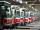Two trams started at the same time from the same place. One tram journey takes 30 minutes and the second 45 minutes to its final stop. How long will trams meet again?
3. Grandfather and grandmotherThe old mother is 5 years younger than the old father. Together they are 153 years old. How many years has each of them?
4. Cars 6At 9:00 am two cars started from the same town and traveled at a rate of 35 miles per hour and the other car traveled at a rate of 40 miles per hour. After how many hours will the cars be 30 miles apart?
5. Class 9AOn the final certificate have one quarter of the class 9A mark "C" of mathematics, seventh mark "C" from the Czech language and two students failed in chemistry. How many students attend class 9A?
6. Train delayThe train should be in the station for 10 minutes. It still has 32 km left. What is the expected delay if the train travels in 2 minutes 3 km except for the last section where it is 2 km in 5 minutes.
7. Round-tripA woman works at a law firm in city A which is about 50 miles from city B. She must go to the law library in city B to get a document. Find how long it takes her to drive​ round-trip if she averages 40 mph.
8. Plum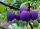On the platter are plum. How many were there if its have to be able to share equally among 8,10 and 12 children?
9. Lcm simpleFind least common multiple of this two numbers: 140 175.
10. Masons1 mason casts 30.8 meters square in 8 hours. How long casts 4 masons 178 meters square?
11. Tram lines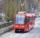Trams of five lines driven at intervals of 5,8,10,12 and 15 minutes. At 12 o'clock come out of the station at the same time. About how many hours again all meet? How many times have earch tram pass for this stop?
12. Balls groups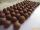Karel pulled the balls out of his pocket and divide it into the groups. He could divide them in four, six or seven, and no ball ever left. How little could be a ball?
13. Raffle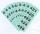In the pool is numbers from 1 to 115. What is the probability that a randomly selected number is not a prime number?
14. Greg and BillGreg is 18 years old. He is 6 less than 4 times Bill's age. How old is Bill?
15. NumberWhat number is 20 % smaller than the number 198?
16. Apples 2James has 13 apples. He has 30 percent more apples than Sam. How many apples has Sam?
17. Bob traveled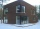Bob traveled 20 meters to Dave's house. He arrived in 3 minutes. What was his average speed for the trip.